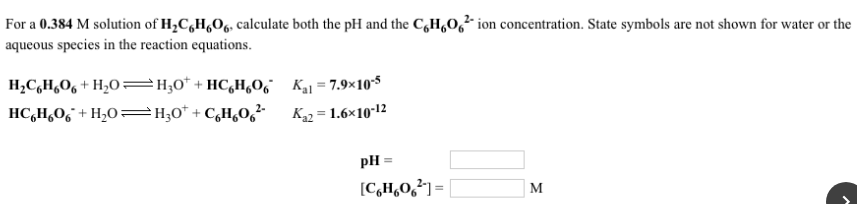# For a 0.384 solution of H2C6H6O6, calculate both the pH and the C6H6O6^2- ion concentration. State symbols are not shown for water or the aqueous species in the reaction equations. H2C6H6O6 + H2O ⇌ H3O^+ + HC6H6O6^- Ka1 = 7.9x10^-5 HC6H6O6^- + H2O ⇌ H3O^+ + C6H6O6^2- Ka2 = 1.6x10^-12